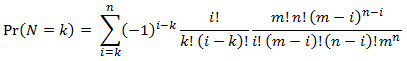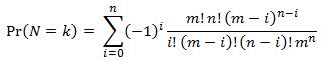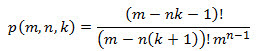## More Birthday Coincidences

Sep 5

Written by: Charles Flock
9/5/2017 9:09 PMA follow-up to our article on the General Birthday Problem, we look at some more interesting calculations relating to birthday ‘coincidences’.

In this article, we look at some more interesting birthday calculations and demonstrate the calculations in SQL Server. These calculations are based on some of the examples in Anirban DasGupta’s 2004 paper, The matching, birthday and the strong birthday problem: a contemporary review. Journal of Statistical Planning and Inference, 130, 377–389.

The strong birthday problem

How many people need to be in a room for there to be a 50% chance that everybody in a group of n individuals has a birthday shared by someone else in the group?

Let N be a random variable which counts the number of unique individuals (those whose birthdays are not shared by anybody else) out of a group of size n; N must lie between 0 and whichever of m or n is smaller.

According to DasGupta, it can be shown that:and using this expression for N = 0 we can calculate the probabilities of everybody in a group of n individuals having a shared birthday in SQL.

Since N = 0, k = 0. This means that we can simplify the equation to:We set m = 365 (we are assuming that birthdays are spread uniformly thought the year and we are ignoring leap years) and we will calculate the probability for a number of different values for n.

Since 365! (m! in the equation) is greater than maximum value that can be calculated in double precision, we are going to use the natural logarithm to do the calculations. This limits the possible values for i to m - 1, since the natural logarithm of zero, ln(0), is undefined.

Here’s one way to approach the calculation using the XLeratorDB FACTLN and SeriesInt functions.

DECLARE @m as float = 365
DECLARE @n as float = 2000

SELECT
seriesvalue as i,
wct.FACTLN(@m)+wct.FACTLN(@n)+(@n-SeriesValue)*LOG(@m-SeriesValue)-(wct.FACTLN(SeriesValue)+wct.FACTLN(@m-SeriesValue)+wct.FACTLN(@n-SeriesValue)+@n*LOG(@m)) as pr
FROM
wct.SeriesInt(0,364,NULL,NULL,NULL)

To come up with the probability, take the exponent of the values calculated in the previous example, multiply the by the first term in the equation, and sum the results.

SELECT
SUM(wct.POWER(-1,i) * EXP(pr)) as pr
FROM (
SELECT
seriesvalue as i,
wct.FACTLN(@m)+wct.FACTLN(@n)+(@n-SeriesValue)*LOG(@m-SeriesValue)-(wct.FACTLN(SeriesValue)+wct.FACTLN(@m-SeriesValue)+wct.FACTLN(@n-SeriesValue)+@n*LOG(@m)) as pr
FROM
wct.SeriesInt(0,364,NULL,NULL,NULL)
)n

This produces the following result.

To reproduce the table from DasGupta’s paper, we could use the following SQL.

DECLARE @m as float = 365

SELECT
n,
SUM(wct.POWER(-1,i) * EXP(pr)) as pr
FROM (
SELECT
X.N,
seriesvalue as i,
wct.FACTLN(@m)+wct.FACTLN(x.n)+(x.n-SeriesValue)*LOG(@m-SeriesValue)-(wct.FACTLN(SeriesValue)+wct.FACTLN(@m-SeriesValue)+wct.FACTLN(x.n-SeriesValue)+x.n*LOG(@m)) as pr
FROM (VALUES
(2000),(2200),(2400),(2600),(2800),(3000),(3063),(3064),(3065),(3200),(3400),(3600),(3800),(4000),(4200),(4400),(4600),(4800),(5000),(5200),(5400),(5600),(5800),(6000),(7000),(8000),(9000))x(n)
CROSS APPLY
wct.SeriesInt(0,364,NULL,NULL,NULL)
)p
GROUP BY
n

This produces the following result.

As you can tell from the table, the answer to the strong birthday problem is 3,064 and in spite of the frightening-looking formula involved, it was quite easy to compute in SQL Server using XLeratorDB.

Near Hits (or Near Misses)

In the case of near hits (or near misses) we are looking at the probability related to 2 people having a birthday, k days apart. When k = 0, this produces the same result as the canonical birthday problem.

DasGupta refers to Abramson and Moler’s 1970 article, “More birthday surprises” published in American Mathematics Monthly, 7 (7), 856-858. Let p(m, n, k) denote the probability that in a group of n people, at least one pair with birthdays within k days of each other’s exists, if there are m equally likely birthdays thenThis can be translated directly into SQL, though I am going to use the natural log of the factorial function rather than the factorial function. Something like this works fine.

--Near Miss Birthday Problem
DECLARE @n as float = 14
DECLARE @k as float = 1
DECLARE @m as float = 365

SELECT 1-EXP(wct.FACTLN(@m-@n*@k-1) - wct.FACTLN(@m-@n*(@k+1)) - (@n-1)*LOG(@m)) as pr

This produces the following result.

So, when there are 14 people in a room, there almost a 54% probability that 2 of them will have a birthday that is 1 day (or less) apart.

In order to determine the room size for a number of different k values, we could run the following SQL. Note that I have simplified the calculation to use the LPERMUT function rather than calling the FACTLN function twice.

--Near Miss Birthday Problem
DECLARE @m as float = 365

;with mycte as (
SELECT
n.SeriesValue as n,
k.seriesValue as k,
1-EXP(wct.LPERMUT(@m-n.SeriesValue*k.seriesValue-1,n.SeriesValue-1)-(n.SeriesValue-1)*LOG(@m)) as p
FROM
wct.SeriesInt(23,1,-1,NULL,NULL)n
CROSS APPLY
wct.SeriesInt(0,7,NULL,NULL,NULL)k
)
SELECT
m.k,
m.n,
m.p
FROM (
SELECT
k,
n,
p,
RANK() OVER (PARTITION BY k ORDER BY p DESC) rnk
FROM
mycte
WHERE
p < 0.5
)n
INNER JOIN
mycte m
ON
n.k = m.k
AND n.n+1 = m.n
WHERE
n.rnk = 1

This produces the following result.

Matched couples

Suppose n couples are invited to a party? What is the likelihood that there are at least two husband-wife pairs such that the husbands have the same birthdays and so do their wives? This calculation works very much the same as with the canonical birthday problem with m = 365 except that we use m2 instead of m.

DECLARE @x as int = 0
DECLARE @m as int = 365
DECLARE @msquare as float = SQUARE(@m)
DECLARE @p as float = 1 - EXP(wct.LPERMUT(@msquare,@x)-@x*LOG(@msquare))

WHILE @p < 0.5
BEGIN
SET @x = @x + 1
SET @p = 1 - EXP(wct.LPERMUT(@msquare,@x)-@x*LOG(@msquare))
END
SELECT @x as N

This produces the following result.

We need 431 couples in a room to have a 50% probability there will be 2 couples where the husbands have the same birthday and the wives have the same birthday.

As you can tell from these ‘coincidences’, I find the math fascinating and informative and very easy to do using a little bit of SQL and a few functions from XLeratorDB. If you are interested in finding out more about doing advanced statistical analysis in SQL Server, you should download the 15-day trial of XLeratorDB and try it out for yourself. It only takes about a minute to download and install and you can just copy the examples from this article right into SSMS to give yourself a quick start.

If you discover that there are functions that you would like added to XLeratorDB, just let us know at support@westclintech.com.

Tags:
Categories:
Location: BlogsThe WestClinTech Blog

## Search Blogs

 KeywordsPhrase

## Blog Archives

Archive
 < September 2023 >
SunMonTueWedThuFriSat
272829303112
3456789
10111213141516
17181920212223
24252627282930
1234567
Monthly
Go

### SupportCopyright 2008-2023 Westclintech LLC         Privacy Policy        Terms of Service